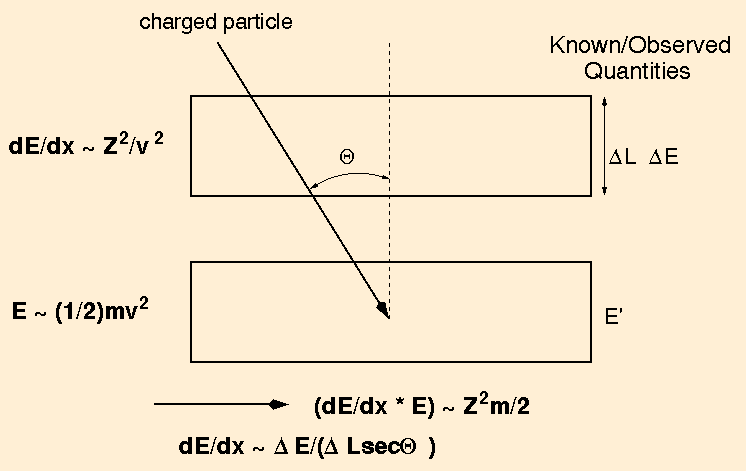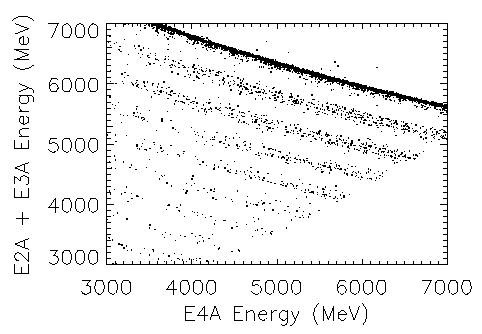## The dE/dx vs. total energy technique for charged particle identification

In a solid-state charged particle telescope such as CRIS or SIS, the only quantities one has available to identify a particle penetrating the telescope are the energy deposited in the various detectors (of known thicknesses) in the "stack" as it comes to rest, and the incident direction (or trajectory) of the particle. The CRIS and SIS stacks consist of several thick silicon detectors placed one above the other; CRIS stacks contain 9 detector elements (some elements consist of pairs of detectors) all having the same thicknesses, while SIS stacks consist of 8 detector elements of varying thicknesses.

Knowledge of the angle of incidence of the particle (relative to the stack normal direction) is obtained through the use of a hodoscope, a device which is capable of determining a particle's trajectory. The hodoscope in CRIS is the Scintillating Optical Fiber Trajectory (SOFT) system, which uses a series of scintillating fibers in alternating directions to observe the trajectory of a particle. Each telescope stack in SIS contains two "matrix detectors", which are thin detectors with charged-particle-sensitive strips on both sides; each side's strips are aligned at 90 degrees to those on the other side, providing event trajectories.This figure describes the general idea behind the method. Using two silicon detectors of known thickness, a particle entering them at a known (thanks to the hodoscope) angle theta will deposit energy DE in the top detector and energy E' in the bottom detector. The derivative dE/dx (energy loss per unit pathlength) can be approximated by the quantity DE/(DL*sec{theta}), where DL is the thickness of the top detector at the particle entry point. The total particle energy is approximated by E', the energy deposited in the bottom detector. This is a reasonable assumption, as it has been found that charged particles tend to lose most of their energy near the end of their range. When the two quantities are multiplied together, the result is approximately equal to Z²M, where Z is the particle charge and M is its mass. As galactic cosmic rays are fully ionized, this is sufficient in principle to uniquely identify the particle, as Z²M is unique for every nucleus we investigate. When a number of such events are collected, DE/(DL*sec{theta}) vs E' data will lie approximately along hyperbolas of constant Z²M. During calibration runs using particle beams entering the telescopes at constant angle, this relationship was verified, as the plot below of DE vs E' demonstrates.### Improvements and Modifications

Several factors have not been taken into account thus far. First, the energy loss relationship, dE/dx ~ Z²/v², does not hold at all energies, and has correction terms which become more important as the particle velocity (kinetic energy) increases. In practice, tabulated or parameterized energy loss vs. particle energy relations are used to refine the more crude charge and mass estimates made by the "hyperbola" method described above.

The second major factor is the existence of "dead layers" in some real silicon detectors. The particle energy DE that is reported does not include the energy deposited in the dead layers; thus the estimate of dE/dx from a particular detector is actually erroneously low. Mass resolution suffers from this effect, but it can be corrected for if the dead layer thickness is known. For the CRIS and SIS detectors, the dead layers have been measured during calibration runs, and are known to within a micron.

Another effect is variations in thickness across a particular detector. The quantity DL can vary across the surface of a real detector of 750 µm (such as are in SIS) by several µm, which will significantly degrade the mass resolution if not corrected for. Thickness "maps" can be used to make this correction. It should be noted that in order to use them, the particle trajectory needs to be known quite well; both the SIS and CRIS hodoscopes are quite capable of determining a particle trajectory sufficiently well to allow such maps to be implemented in the data analysis.

Analysis of SIS & CRIS data is helped by the fact that redundant estimates of particle charge and mass can be made (except for particles stopping in the second stack detector). For example, the stopping detector and the one above it could have their energy signals added together to provide an estimate of E', with the detector above those providing an estimate of DE. For a particle stopping in the n-th detector from the top, there are thus (n-1) independent estimates of Z and M that can be generated. Requiring consistency between these estimates can be used to reject events that have undergone interactions in the silicon wafers themselves, for example.